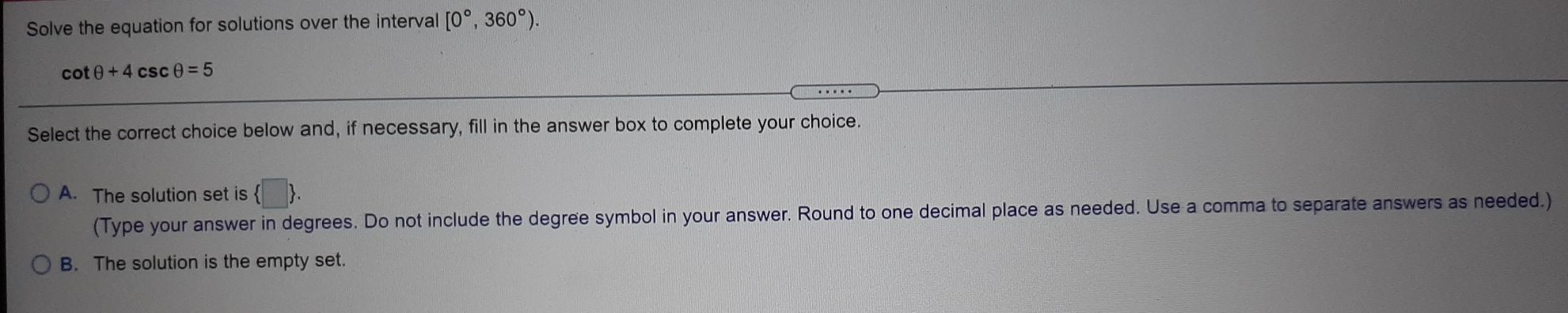### Still have math questions?

Algebra
QuestionSolve the equation for solutions over the interval $$[ 0 ^ { \circ } , 360 ^ { \circ } )$$ . $$\cot \theta + 4 \csc \theta = 5$$ .

Select the correct choice below and, if necessary, fill in the answer box to complete your choice.

A. The solution set is $$\square$$ . (Type your answer in degrees. Do not include the degree symbol in your answer. Round to one decimal place as needed. Use a comma to separate answers as needed.)

B. The solution is the empty set.